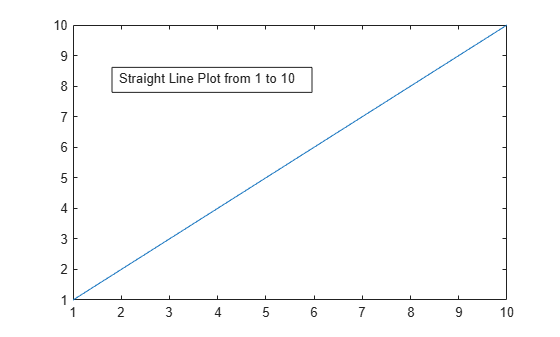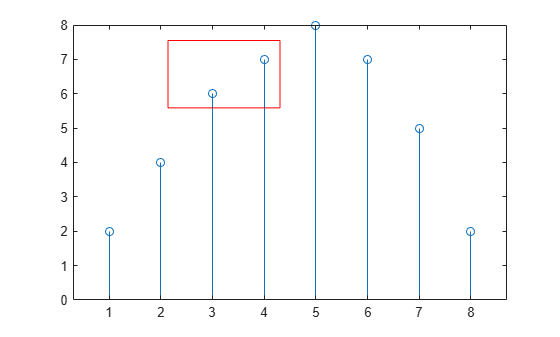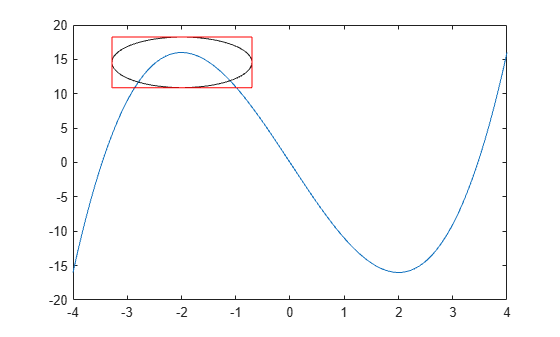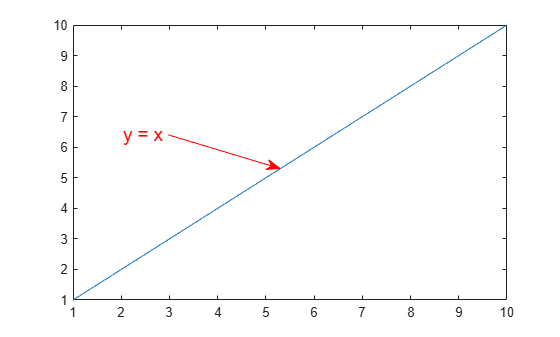# annotation

Create annotations

## Syntax

``annotation(lineType,x,y)``
``annotation(lineType)``
``annotation(shapeType,dim)``
``annotation(shapeType)``
``annotation(___,Name,Value)``
``annotation(container,___)``
``an = annotation(___)``

## Description

example

````annotation(lineType,x,y)` creates a line or arrow annotation extending between two points in the current figure. Specify `lineType` as `'line'`, `'arrow'`, `'doublearrow'`, or `'textarrow'`. Specify `x` and `y` as two-element vectors of the form ```[x_begin x_end]``` and ```[y_begin y_end]```, respectively.```
````annotation(lineType)` creates the annotation in the default position between the points `(0.3,0.3)` and `(0.4,0.4)`.```

example

````annotation(shapeType,dim)` creates a rectangle, ellipse, or text box annotation with a particular size and location in the current figure. Specify `shapeType` as `'rectangle'`, `'ellipse'`, or `'textbox'`. Specify `dim` as a four-element vector of the form `[x y w h]`. The `x` and `y` elements determine the position and the `w` and `h` elements determine the size.```
````annotation(shapeType)` creates the annotation in the default position so that the lower left corner is at `(0.3,0.3)` and the width and height are both `0.1`.```

example

````annotation(___,Name,Value)` creates the annotation and specifies properties as name-value pair arguments. Different types of annotations support different properties. You can specify properties with any of the input argument combinations in the previous syntaxes.```
````annotation(container,___)` creates the annotation in the figure, uipanel, or uitab specified by `container`, instead of in the current figure.```

example

````an = annotation(___)` returns the annotation object. The type of object returned depends on first input argument. Use `an` to modify properties of the object after it is created. You can specify an output argument with any of the previous syntaxes. ```

## Examples

collapse all

Create a simple line plot and add a text arrow to the figure. Specify the text arrow location in normalized figure coordinates, starting at the point `(0.3,0.6)` and ending at `(0.5,0.5)`. Specify the text description by setting the `String` property.

```figure plot(1:10) x = [0.3 0.5]; y = [0.6 0.5]; annotation('textarrow',x,y,'String','y = x ')```Create a simple line plot and add a text box annotation to the figure. Specify the text description by setting the `String` property. Force the box to fit tightly around the text by setting the `FitBoxToText` property to `'on'`.

```figure plot(1:10) dim = [.2 .5 .3 .3]; str = 'Straight Line Plot from 1 to 10'; annotation('textbox',dim,'String',str,'FitBoxToText','on');```Create a text box annotation without setting the `FitBoxToText` property. The text box uses the specified width and height and wraps text as needed.

```figure plot(1:10) dim = [.2 .5 .3 .3]; str = 'Straight Line Plot from 1 to 10'; annotation('textbox',dim,'String',str)```Create a text box annotation with multiline text by setting the `String` property to a cell array. Each element of the cell array displays on a separate line. Force the box to fit tightly around the text by setting the `FitBoxToText` property to `'on'`.

```figure plot(1:10) dim = [0.2 0.5 0.3 0.3]; str = {'Straight Line Plot','from 1 to 10'}; annotation('textbox',dim,'String',str,'FitBoxToText','on');```Create a stem plot and add a rectangle annotation to the figure. Change the color of the rectangle outline by specifying the `Color` property.

```figure data = [2 4 6 7 8 7 5 2]; stem(data) dim = [.3 .68 .2 .2]; annotation('rectangle',dim,'Color','red')```Add a second rectangle annotation to the figure. Specify the fill color by setting the `FaceColor` property. Add transparency by setting the `FaceAlpha` property to a value between 0 (completely transparent) and 1 (completely opaque).

```dim2 = [.74 .56 .1 .1]; annotation('rectangle',dim2,'FaceColor','blue','FaceAlpha',.2)```Create a simple line plot and add an ellipse annotation to the figure. Specify `dim` as the size and location of the smallest rectangle that encloses the ellipse.

```figure x = linspace(-4,4); y = x.^3 - 12*x; plot(x,y) dim = [.2 .74 .25 .15]; annotation('ellipse',dim)```Draw a red rectangle using the same dimensions to show how the ellipse fills the rectangular area.

`annotation('rectangle',dim,'Color','red')`Create a simple line plot. Then, add a bent arrow to the graph by combining a line and an arrow annotation.

```figure plot(1:10) xl = [.3 .3]; yl = [.3 .4]; annotation('line',xl,yl) xa = [.3 .4]; ya = [.4 .4]; annotation('arrow',xa,ya)```Add a text arrow to a figure and return the annotation text arrow object, `a`.

```figure plot(1:10) x = [0.3,0.5]; y = [0.6,0.5]; a = annotation('textarrow',x,y,'String','y = x ');```Modify properties of the annotation text arrow using `a`. For example, change the color to red and the font size to 14 points.

```a.Color = 'red'; a.FontSize = 14;```See the annotation property pages for a list of properties for each type of annotation.

## Input Arguments

collapse all

Type of line annotation, specified as one of these values.

ValueType of ObjectExample
`'line'`Annotation line`annotation('line',[.1 .2],[.1 .2])`
`'arrow'`Annotation arrow`annotation('arrow',[.1 .2],[.1 .2])`
`'doublearrow'`Annotation double arrow`annotation('doublearrow',[.1 .2],[.1 .2])`
`'textarrow'`

Annotation text arrow. To add text to the tail end of the text arrow, use the `String` property.

```annotation('textarrow',[.1 .2],[.1 .2],'String','my text')```

Type of shape annotation, specified as one of these values.

ValueType of ObjectExample
`'rectangle'`Annotation rectangle`annotation('rectangle',[.2 .3 .4 .5])`
`'ellipse'`Annotation ellipse`annotation('ellipse',[.2 .3 .4 .5])`
`'textbox'`

Annotation text box. To specify the text, set the `String` property. To automatically adjust the dimensions of the box to fit closely around the text, set the `FitBoxToText` property to `'on'`.

`annotation('textbox',[.2 .3 .4 .5],'String','my text','FitBoxToText','on')`

Beginning and ending x-coordinates, specified as a two-element vector of the form `[x_begin x_end]`. Together the `x` and `y` input arguments determine the endpoints of the line, arrow, double arrow, or text arrow annotation. The annotation extends from the point (`x_begin`, `y_begin`) to (`x_end`, `y_end`).

By default, the units are normalized to the figure. The lower left corner of the figure maps to `(0,0)` and the upper right corner maps to `(1,1)`. To change the units, use the `Units` property.

Example: `x = [.3 .5]`

Beginning and ending y-coordinates, specified as a two-element vector of the form `[y_begin y_end]`. Together the `x` and `y` input arguments determine the endpoints of the line, arrow, double arrow, or text arrow annotation. The annotation extends from the point (`x_begin`, `y_begin`) to (`x_end`, `y_end`).

By default, the units are normalized to the figure. The lower left corner of the figure maps to `(0,0)` and the upper right corner maps to `(1,1)`. To change the units, use the `Units` property.

Example: `y = [.3 .5]`

Size and location, specified as a four-element vector of the form `[x y w h]`. The first two elements specify the coordinates of the lower left corner of the text box, rectangle, or ellipse with respect to the lower left corner of the figure. The second two elements specify the width and height of the annotation, respectively.

If you are creating an ellipse, then `dim` is the size and location of the smallest rectangle that encloses the ellipse.

By default, the units are normalized to the figure. The lower left corner of the figure maps to `(0,0)` and the upper right corner maps to `(1,1)`. To change the units, use the `Units` property

Example: `dim = [.3 .4 .5 .6]`

Target for annotation, specified as a figure, uipanel, or uitab object. For example, to add an annotation to a specific figure, specify the figure object as the first input argument to the function.

```f = figure; annotation(f,'line',[.1 .2],[.2 .3])```

### Name-Value Arguments

Specify optional comma-separated pairs of `Name,Value` arguments. `Name` is the argument name and `Value` is the corresponding value. `Name` must appear inside quotes. You can specify several name and value pair arguments in any order as `Name1,Value1,...,NameN,ValueN`.

Example: `annotation('rectangle',[.5 .5 .1 .1],'EdgeColor','r')` creates a rectangle annotation with a red outline.

Each type of annotation object supports a different set of properties. For a full list of properties and descriptions for each type, see the associated property page.

## Output Arguments

collapse all

Annotation object, returned as a scalar. The type of annotation object returned depends on the first input argument.

First Input ArgumentType of Object ReturnedProperty Page
`'line'`LineLine Properties
`'arrow'`ArrowArrow Properties
`'doublearrow'`Double arrowDoubleEndArrow Properties
`'textarrow'`Text arrowTextArrow Properties
`'textbox'`Text boxTextBox Properties
`'rectangle'`RectangleRectangle Properties
`'ellipse'`EllipseEllipse Properties

collapse all

### Compatibility Considerations

Starting in R2014b, annotations cannot cross uipanel boundaries. Instead, they clip at the boundaries. Previous versions of MATLAB® allow annotations to extend into (or out of) the boundaries. To display an annotation within a specific figure, uipanel, or uitab, use the `container` input argument.

### Topics

Introduced before R2006a